# 航空公司客户价值分析

## 项目目标

• 借助航空公司客户数据，对客户进行分类
• 对不同的客户类别进行特征分析，比较不同类别客户的客户价值
• 对不同价值的客户类别提供个性化服务，制定相应的营销策略

### 了解客户价值分析

• 公司收入的80%来自顶端的20%的客户。
• 20%的客户其理论率100%。
• 90%以上的收入来自现有客户。
• 大部分的营销预算经常被用在非现有客户上。
• 5%至30%的客户在客户金字塔中具有升级潜力。
• 客户金字塔中客户升级2%，意味着销售收入增加10%，利润增加50%。

### 模型

RFM模型：最近消费时间间隔（Recency）、消费频率（Frequency）和消费金额（Monetary）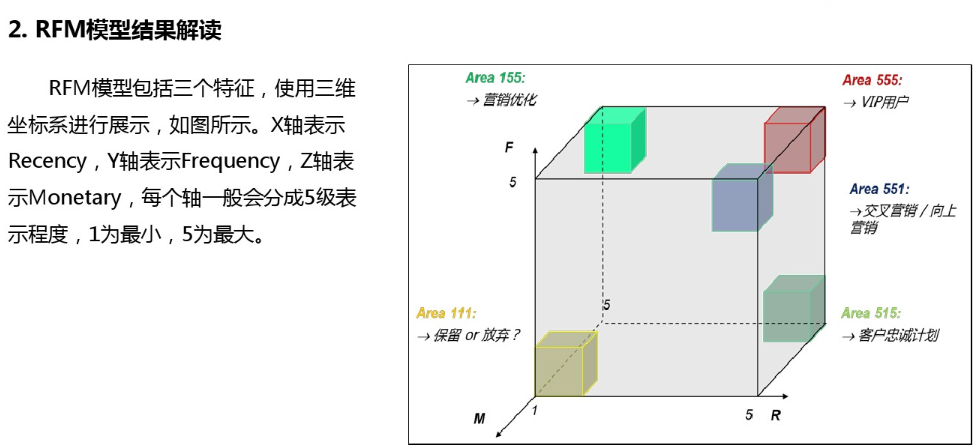LRFMC模型

• L——会员入会时间距观测窗口结束的月数
• R——客户最后一次乘坐飞机距观测窗口结束的月数
• F——客户在观测窗口内乘坐飞机的次数
• M——客户在观测窗口内的总飞行里程
• C——客户在观测窗口内乘坐的舱位对应折扣系数的平均值

#### 属性构造

• L=LOAD_TIME – FFP_DATE(观测窗口的结束时间 – 入会时间)
• R=LAST_TO_END(最后一次乘坐飞机距观测窗口结束的时长)
• F=FLIGHT_COUNT(观测窗口内的飞行次数)
• M=SEG_KM_SUM(观测窗口内的总飞行里程)
• C=AVG_DISCOUNT(平均折扣率)

## 数据预处理

### 数据预分析

``````explore['null'] = len(data) - explore['count']
explore = explore[['null', 'max', 'min']]
explore.columns = [['空值数','最大值','最小值']]
explore
``````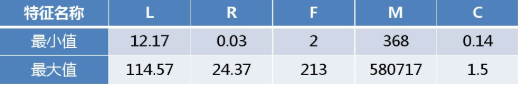### 数据清洗

• 票价为空
• 票价为0，平均折扣率不为0，总飞行里程数大于0

``````# 数据清洗, 保留票价非空
data = data[data['SUM_YR_1'].notnull() & data['SUM_YR_2'].notnull()]

# 只保留票价非零, 或者平均折扣率为0的值
index1 = data['SUM_YR_1'] != 0
index2 = data['SUM_YR_2'] != 0
index3 = data['avg_discount'] == 0
data = data[index1 | index2 | index3]
``````

### 数据标准化

``````data = (df - df.mean(axis=0))/(df.std(axis=0))
data.columns = ['Z' + i for i in df.columns]
``````

## 聚类算法

`from sklearn.cluster import KMeans`

### 基本概念

K-Means聚类算法是一种基于质心的划分方法，输入聚类个数k，以及包含n个数据对象的数据库，输出满足误差平方和最小标准的k个聚类。算法步骤如下：

1. 从n个样本数据中随机抽取k个对象作为初始的聚类中心
2. 分别计算每个样本到各个聚类质心的距离，将样本分配到距离最近的那个聚类中心类别中
3. 所有样本分配完成后，重新计算k个聚类的中心
4. 与前一次计算得到的k个聚类中心比较，如果聚类中心发生变化，转（2），否者转（5）
5. 当质心不发生变化时，停止并输出聚类结果
``````def __init__(self, n_clusters=8, init='k-means++',
n_init=10, max_iter=300,tol=1e-4,precompute_distances='auto',
verbose=0, random_state=None, copy_x=True, n_jobs=1):
``````

（1）`n_clusters`：要分成的簇数也是要生成的质心数

``````n_clusters : int, optional, default: 8
``````

The number of clusters to form as well as the number of centroids to generate.

（2）init:初始化质心

kmeans++：种子点选取的第二种方法。

``````kmedoids(PAM,Partitioning Around Medoids)
``````

``````init : {'k-means++', 'random' or an ndarray}
``````

Method for initialization, defaults to ‘k-means++’:
‘k-means++’ : selects initial cluster centers for k-mean clustering in
a smart way to speed up convergence. See section Notes in k_init for more details.

（3）`n_init`: 设置选择质心种子次数，默认为10次。返回质心最好的一次结果（好是指计算时长短）

``````n_init : int, default: 10
``````

Number of time the k-means algorithm will be run with different centroid seeds.
The final results will be the best output of n_init consecutive runs in terms of inertia.

（4）`max_iter`：每次迭代的最大次数

``````max_iter : int, default: 300
``````

Maximum number of iterations of the k-means algorithm for a
single run.

（5）`tol`: 容忍的最小误差，当误差小于tol就会退出迭代（算法中会依赖数据本身）

Relative tolerance with regards to inertia to declare convergence

（6）`precompute_distances`: 这个参数会在空间和时间之间做权衡，如果是`True` 会把整个距离矩阵都放到内存中，`auto` 会默认在数据样本大于featurs*samples 的数量大于`12e6` 的时候`False`,`False`时核心实现的方法是利用Cpython 来实现的

Precompute distances (faster but takes more memory).
‘auto’ : do not precompute distances if n_samples * n_clusters > 12 million. This corresponds to about 100MB overhead per job usingdouble precision.

（7）`verbose`:是否输出详细信息

verbose : boolean, optional
Verbosity mode.

（8）`random_state`： 随机生成器的种子 ，和初始化中心有关

random_state : integer or numpy.RandomState, optional
The generator used to initialize the centers. If an integer is given, it fixes the seed. Defaults to the global numpy random number generator.

（9）`copy_x``bool``scikit-learn` 很多接口中都会有这个参数的，就是是否对输入数据继续copy 操作，以便不修改用户的输入数据。这个要理解Python 的内存机制才会比较清楚。

When pre-computing distances it is more numerically accurate to center the data first. If copy_x is True, then the original data is not modified. If False, the original data is modified, and put back before the function returns, but small numerical differences may be introduced by subtracting and then adding the data mean.

（10）`n_jobs`: 使用进程的数量，与电脑的CPU有关

The number of jobs to use for the computation. This works by computing
each of the n_init runs in parallel.
If -1 all CPUs are used. If 1 is given, no parallel computing code is used at all, which is useful for debugging. For n_jobs below -1,(n_cpus + 1 + n_jobs) are used. Thus for n_jobs = -2, all CPUs but one
are used.

（二）输出参数：

（1）`label_`:每个样本对应的簇类别标签

（2）`cluster_centers_`：聚类中心

``````from sklearn.cluster import KMeans
k = 5
kmodel = KMeans(n_clusters = k, n_jobs = 4)
kmodel.fit(data)   # 训练数据
``````
``````# 查看聚类中心
kmodel.cluster_centers_
``````
``````array([[ 0.05413636, -0.00294837, -0.22884398, -0.23289087,  2.18362792],
[ 1.16107019, -0.37746281, -0.0866874 , -0.09471551, -0.15729059],
[-0.31407344,  1.68560841, -0.57386122, -0.53668581, -0.1753762 ],
[ 0.48302008, -0.79948458,  2.48279483,  2.42422366,  0.30947997],
[-0.7000998 , -0.4153057 , -0.16094143, -0.16073752, -0.25434129]])
``````

### 分析聚类结果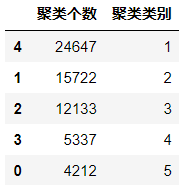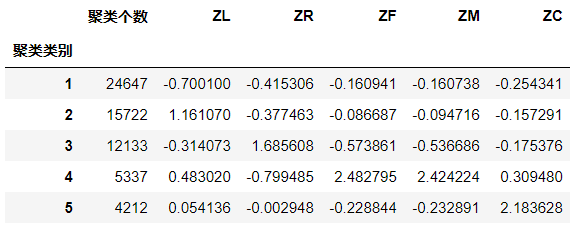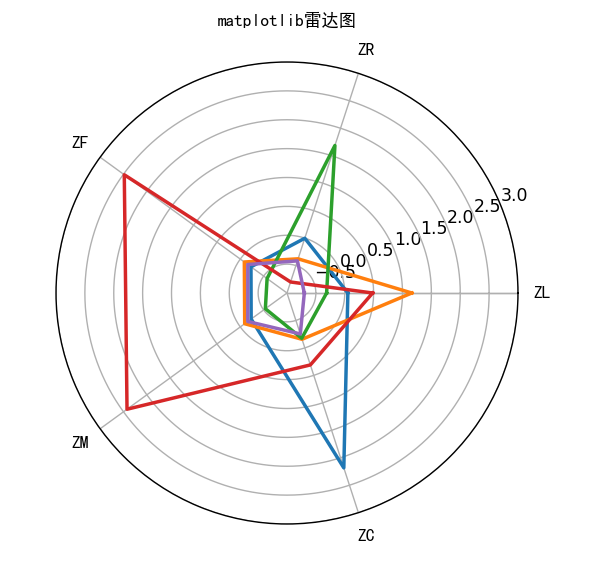### 模型应用

• 对于数量极少的客户群4，进行一对一精准营销。
• 对于数量极少的客户群5，实行里程数兑换机票。
• 对于数量较大的客户群2，提供会员升级提醒服务。
• 积极和非航空类企业合作，顾客在合作企业消费也可获得本航空公司奖励，增加客户与公司的联系。